# JEE Main Heat Transfer Previous Year Questions with Solutions

According to thermodynamic systems, heat transfer is defined as, “The movement of heat across the border of the system due to a difference in temperature between the system and its surroundings”.

The motion of atoms and molecules in a matter causes the heat or thermal energy. Any matter which is made up of atoms and molecules has the ability to transfer heat. The heat energy will be more when the motion of the molecules is more. However, talking about heat transfer, it is nothing but the process of transfer of heat from a high-temperature body to a low temperature one. Heat transfer will cause a change in internal energy. Internal energy is the total sum of the potential energy and kinetic energy of the system. The change in internal energy depends on the heat gained or lost by the system, provided the pressure is constant.

Related Articles:

Heat Transfer and Calorimetry

Conduction

## JEE Main Previous Year Solved Questions on Heat Transfer

Q1: A gas can be taken from A to B via two different processes ACB and ADB. When P C B A D V path ACB is used 60 J of heat flows into the system and 30 J of work is done by the system. If path ADB is used, work done by the system is 10 J. The heat flow into the system in path ADB is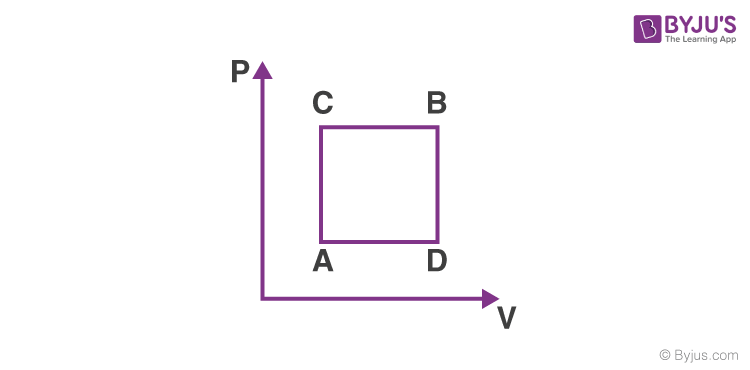(a) 100 J

(b) 80 J

(c) 40 J

(d) 20 J

Solution

ΔQ = ΔU + ΔW

ΔU = ΔQ – ΔW

60 – 30 = ΔQ – 10 Q = 40 J

Q2: In a process, temperature and volume of one mole of an ideal monoatomic gas are varied according to the relation VT = K, where K is a constant. In this process, the temperature of the gas is increased by T. The amount of heat absorbed by gas is given by (R is gas constant)

(a) (2K/3)ΔT

(b) ½RΔT

(c) (3/2)RΔT

(d) (½)KRΔT

Solution

VT = K,

V(PV/R) = K

PV2 = constant

For a polytropic process

C = (R/1-x) + Cv = (R/1-2) + 3R/2 = R/2

ΔQ = n C T = (½) RT

Q3: A cylinder with a fixed capacity of 67.2 lit contains helium gas at STP. The amount of heat needed to raise the temperature of the gas by 20°C is [Given that R = 8.31 J mol–1 K–1]

(a) 350 J

(b) 700 J

(c) 748 J

(d) 374 J

Solution

Number of moles of gas, n = (67.2 /22.4) = 3 mol

ΔQ = nCvΔT = 3 x (3/2)R x ΔT = 3 x (3/2) x (8.31) x 20 = 747.9 =748 J

Q4: 200 g water is heated from 40° C to 60°C. Ignoring the slight expansion of water, the change in its internal energy is close to (Given specific heat of water = 4184 J/kg/K)

(a) 167.4 kJ

(b) 8.4 kJ

(c) 4.2 kJ

(d) 16.7 kJ

Solution

For isochoric process,

ΔU = Q = msΔT Here, m = 200 g = 0.2 kg, s = 4184 J/kg/K

ΔT = 60°C – 40°C = 20 °C = 20 K

ΔU = 0.2 × 4184 20 = 16736 J = 16.7 kJ

Q5: A diatomic gas with rigid molecules does 10 J of work when expanded at constant pressure. What would be the heat energy absorbed by the gas, in this process?

(a) 25 J

(b) 30 J

(c) 35 J

(d) 40 J

Solution:

Given that the process is isobaric.

Therefore, heat energy absorbed by the gas is ΔQ = nCPT …(1)

Also, work done by the gas is W = nRT = 10 J (given)…….(2)

Since, CP = (7/2) R for a diatomic gas

ΔQ = n(7/2) RΔT (Using 1)

ΔQ =(7/2)nRΔT =( 7/2) x 10 (Using 2)

ΔQ = 35 J

Q6: A long metallic bar is carrying heat from one of its ends to the other end under steady-state. The variation of temperature θ along the length x of the bar from its hot end is best described by which of the following figures?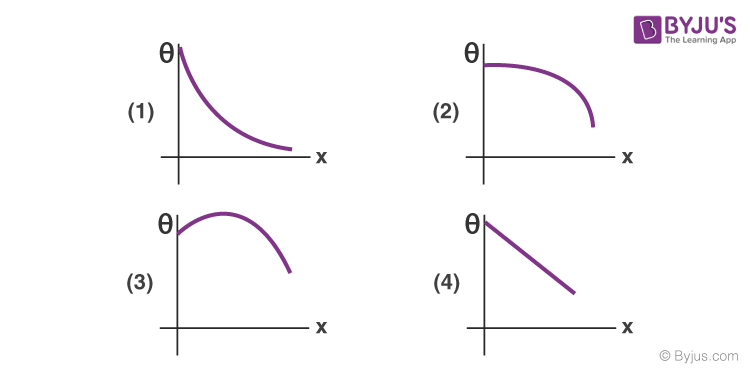Solution:

The heat flow rate is given by

dQ/dt = KA(θ1 – θ)

θ1 – θ = (x/KA)(dQ/dt) ⇒ θ = θ1 – (x/KA)(dQ/dt)

θ1 = Temperature at the hot end

θ = Temperature at a distance x from hot end

The above equation can be represented graphically by

Q7: If a piece of metal is heated to temperature θ and then allowed to cool in a room which is at a temperature θ the graph between the temperature T of the metal and time t will be closed to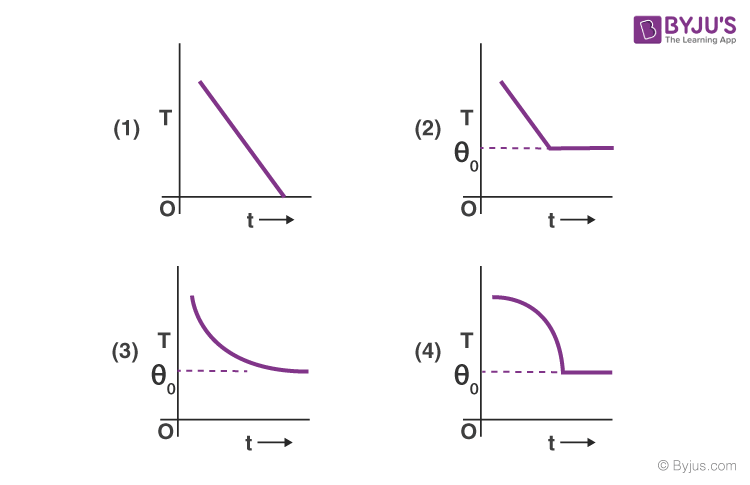Solution:

According to Newton’s law of cooling, the rate of loss of heat is proportional to the difference in temperature of the body and the surroundings

dQ/dt = k(T2 – T1)——-(1)

dQ/dt = msdT2/dt——–(2)

From (1) and (2) we get

msdT2/dt = k(T2 – T1)

dT2 /(T2 – T1) = (-k/ms)dt = -Kdt [K = k/ms]

On integrating we get

log e(T2 – T1) = -Kt + C

T2 = T1 + C’e-Kt (where, C’ = ec]

This variation is represented by graph (c)

Q8: A heat source at T=103 K is connected to another heat reservoir at T = 102 K by a copper slab which is 1mm thick. Given that the thermal conductivity of copper is 0.1 WK-1m-1, the energy flux through it in the steady-state is

1. 90 Wm-2
2. 120 Wm-2
3. 65 Wm-2
4. 200 Wm-2

Solution: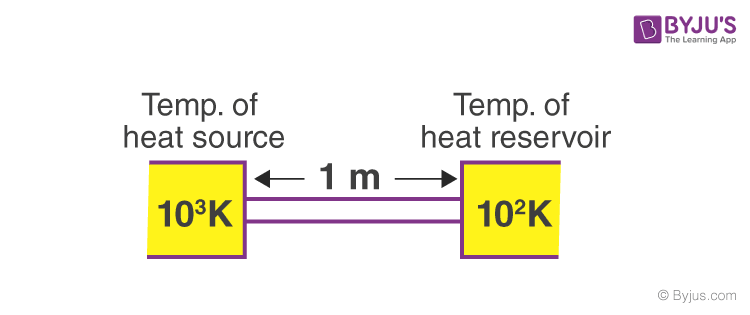(dQ/dt) = kAΔT/l

Energy flux, $\frac{1}{A}\left ( \frac{dQ}{dt} \right )=\frac{k\Delta T}{l}$

= (0.1)(900)/1 = 90 W/m2

Q9: Two materials having coefficients of thermal conductivity ‘3K’ and ‘K’ and thickness’ and ‘3d’ respectively, are joined to form a slab as shown in the figure. The temperature of the outer surface is ‘θ2’ and ‘θ1’ respectively, (θ2 θ1). The temperature at the interface is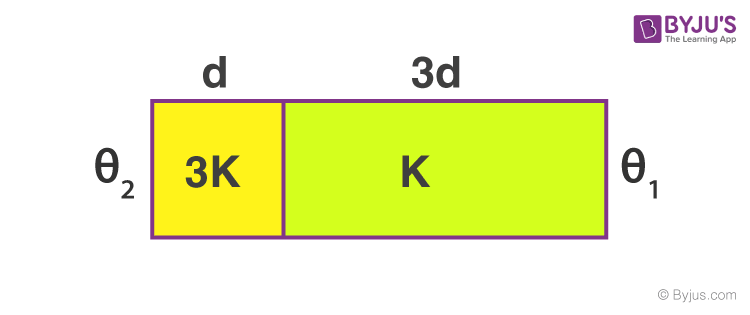1. 1/10) + (9θ2/10)
2. 2 + θ1)/2
3. 1/6) + (5θ2/6)
4. 1/3) + (2θ2/3)

Solution

H1= H2θ2

(3k)A[(θ2 – θ)/d] = kA[(θ – θ1)/3d]

θ = (θ1/10) + (9θ2/10)

Q10: A cylinder of radius R is surrounded by a cylinder shell of inner radius 2R. The thermal conductivity of the material of the cylinder is K1 and that of the outer cylinder is K2. Assuming no loss of heat, the effective thermal conductivity of the system for heat flowing along the length of the cylinder is

1. (K1 + K2)/2
2. K1 + K2
3. (2K1 + 3K2)/5
4. (K1 + 3K2)/4

Solution: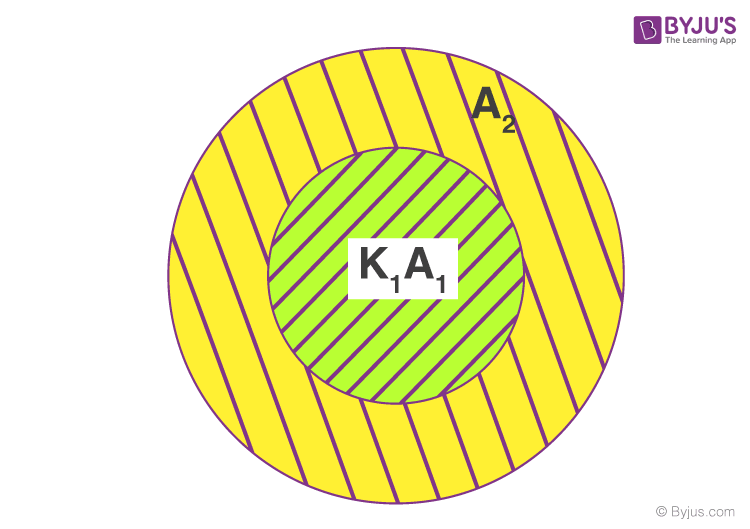Keq = (K1A1 + K2A2)/(A1 + A2)

Keq = K1πR2 + K2[π(2R)2 – πR2]/π(2R)2

= (K1+ 3K2)/4

Q11: Heat given to a body which raises its temperature by 1°C is

(a) water equivalent

(b) thermal capacity

(c) specific heat

Solution: (b) thermal capacity

Q12: The temperature of the two outer surfaces of a composite slab, consisting of two materials having coefficients of thermal conductivity K and 2K and thickness x and 4x, respectively are T2 and T1 (T2 > T1). The rate of heat transfer through the slab, in a steady-state, is [A(T2-T1)K/x]f, with f equal to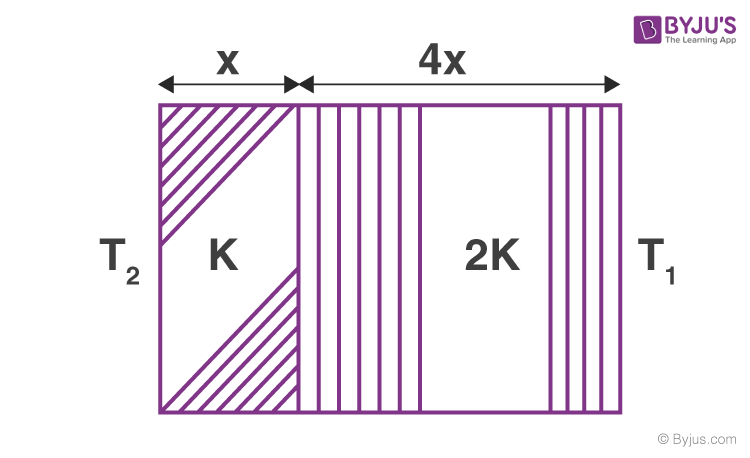1. 1
2. ½
3. 1/3

Solution

For the first surface, Q1 = KA(T2 -T)t/x

For second surface, Q2 = (2K)A(T -T)t/(4x)

At steady state, Q2 = Q1⇒ KA(T2 -T)t/x = (2K)A(T -T1)t/(4x)

Or 2(T2 -T) = (T -T1)

T = (2T2+ T1)/3

Q1 = KA/x (T2 – [(2T2+T1)/3])t

$\left [ \frac{A(T_{2}-T_{1})K}{x} \right ]f = \frac{KA}{x}\left [ \frac{T_{2}-T_{1}}{3} \right ]\times 1$

f = ⅓

Q13: According to Newton’s law of cooling, the rate of cooling of a body is proportional to (ΔӨ)n, where is the difference of the temperature of the body and the surroundings, and n is equal to

(a) two

(b) three

(c) four

(d) one

Solution:

According to Newton’s law of cooling, the rate of cooling is proportional to ΔӨ (ΔӨ ) n = (ΔӨ) or n = 1.

Q14: When an ideal diatomic gas is heated at constant pressure, the fraction of the heat energy supplied which increases the internal energy of the gas is

(a) 2/5

(b) 3/5

(c) 3/7

(d) 5/7

Solution:

ΔU = nCvΔT

ΔQ = nCpΔT

Therefore, ΔU/ΔQ = nCvΔT/nCpΔT = Cv/Cp = 1/γ = 5/7

Q15: 70 calories of heat are required to raise the temperature of 2 moles of an ideal gas at constant pressure from 30°C to 35°C. The amount of heat required (in calorie) to raise the temperature of the same gas through the same range (30°C to 35°C) at constant volume is

1. 30
2. 50
3. 70
4. 90

Solution

Q = nCpdT

Cp = Q/ndT = 70/2x(35 – 30)

Cp = 70/(2×5)

Cp = 7 cal/mol x K

Now, Cv = Cp – R

Cv = 7-2 =5 cal/mol x K

Q’ = nCvdT = 2 x 5 x 5 =50 cal